# Dupont Analysis Excel Template

The DuPont system of analysis is an approach that is used a lot by analysts to analyze the Return on Equity (ROE). The approach breaks down the return on equity into different parts. This way, we can analyze the impact of turnover, profit margins, and leverage on ROE. On this page, we discuss two versions of the approach, the original ‘3 step Dupont analysis‘ and the ‘5 step Dupont analysis‘. Finally, we discuss an example and provide a DuPont analysis Excel template for download.

## Dupont System of Analysis

First, we begin with discussing the original DuPont ratio. To keep things simple, we first start with the ROE formula: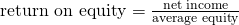Next, we multiple the ROE formula with (revenue/revenue) and (average total assets/average total assets). Since the numerator and denominator are the same in both ratios, this equivalent to multiplying ROE by 1. At the same time, we also rearrange the terms: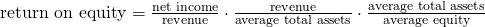The formula above is the simple version of the the DuPont formula. It shows that ROE can be composed in three parts: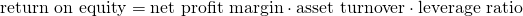This formula is the most important equation  in ratio analysis. If ROE is low, the formula shows what is causing the low ROE. It can be due to low asset turnover, a poor profit margin, or too little leverage.

Next, we discuss the extended (5-stage) DuPont Equation. The approach is similar to the one above. But here we multiply the ROE ratio with two additional ratios; (EBIT/EBIT) and (EBT/EBT). That is, Earnings before Interest and Taxes (EBIT) and Earnings Before Taxes (EBT). Again, we arrange a bit in the process. Then, the formula becomes: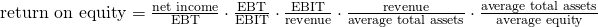In words, the different ratios included above are are: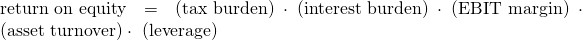The advantage of the DuPont analysis when using the advanced approach is that the advanced approach illustrates that more leverage is not always a good thing. In particular, more leverage not only increase ROE, it can also decrease ROE! This is because with more debt and leverage, the interest burden also increases!

From the formula it is also clear that higher taxes will always lower the ROE.

## Summary

We discussed the DuPont ratio, which is used by analysts to decompose ROE. It is arguably the most important ratio in ratio analysis.

### Dupont model Excel template

How to do DuPont analysis in Excel? The following Excel is a DuPont calculator that implements a DuPont Analysis example: DuPont Analysis Excel Template.